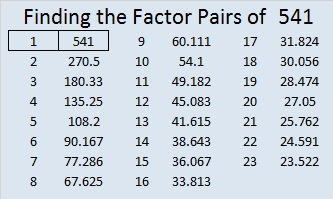# 541 and Level 5

541 = 21² + 10² = 441 + 100

541 is the hypotenuse of the primitive Pythagorean triple 341-420-541

And finally, after the longest string of composite numbers so far, 541 is quite notably a prime number. In fact, it is the 100th prime number.Print the puzzles or type the solution on this excel file: 12 Factors 2015-06-29

—————————————————————————————————

• 541 is a prime number.
• Prime factorization: 541 is prime.
• The exponent of prime number 541 is 1. Adding 1 to that exponent we get (1 + 1) = 2. Therefore 541 has exactly 2 factors.
• Factors of 541: 1, 541
• Factor pairs: 541 = 1 x 541
• 541 has no square factors that allow its square root to be simplified. √541 ≈ 23.25940 66 99 22 601 44How do we know that 541 is a prime number? If 541 were not a prime number, then it would be divisible by at least one prime number less than or equal to √541 ≈ 23.3. Since 541 cannot be divided evenly by 2, 3, 5, 7, 11, 13, 17, 19, or 23, we know that 541 is a prime number.

—————————————————————————————————This site uses Akismet to reduce spam. Learn how your comment data is processed.